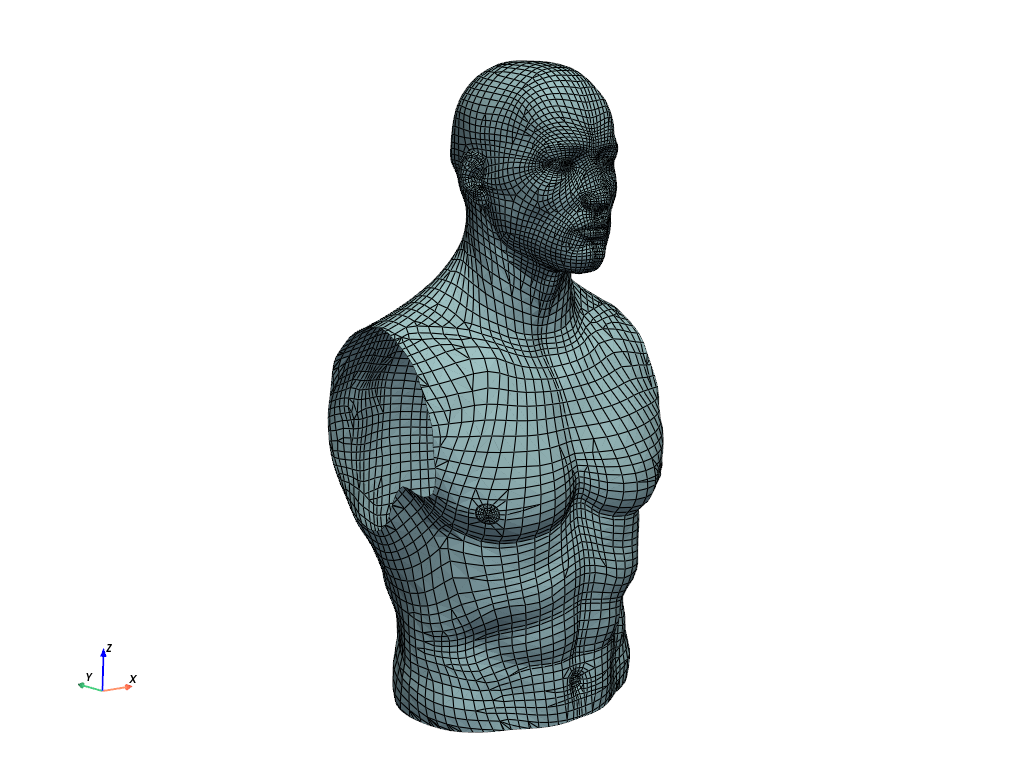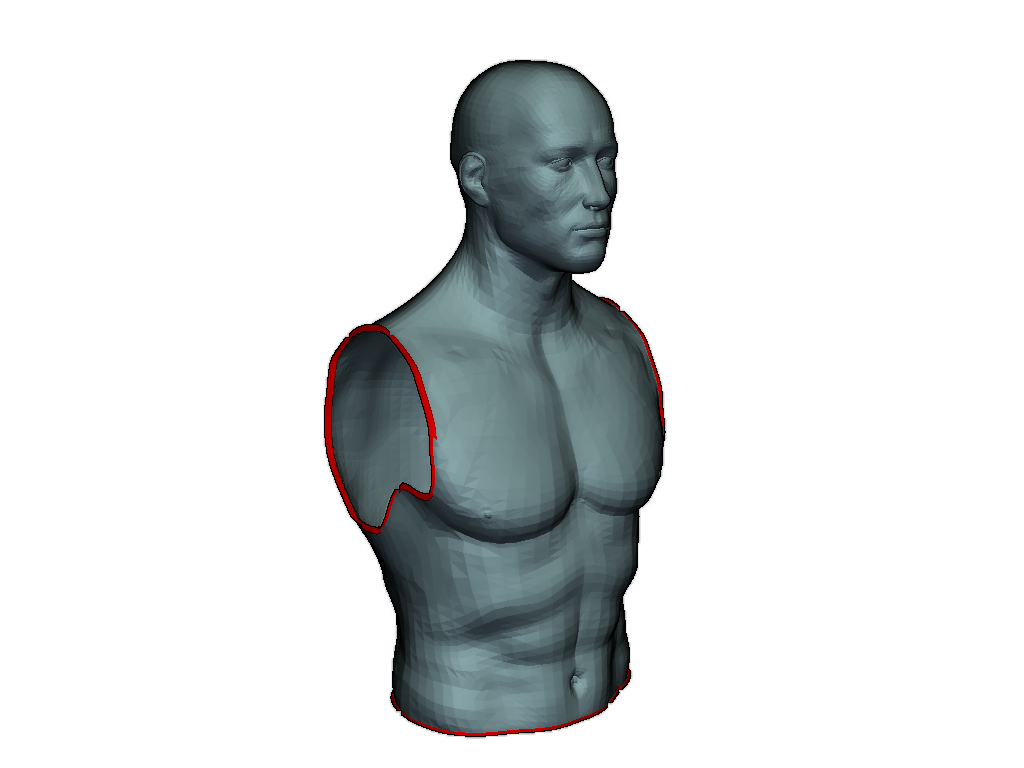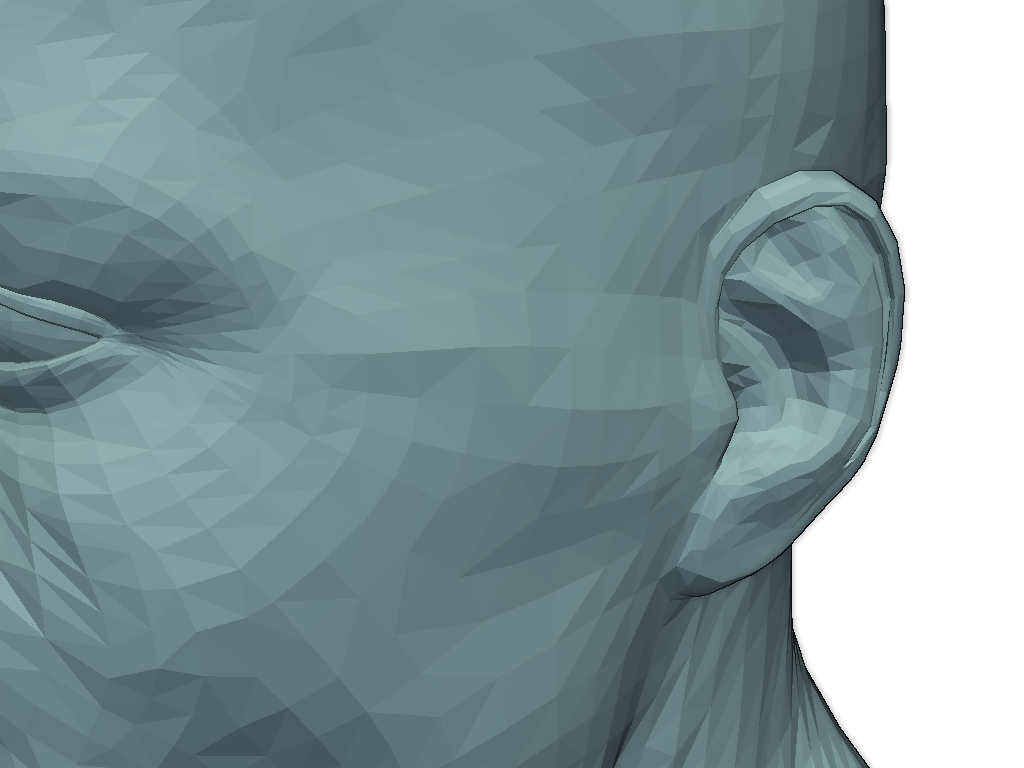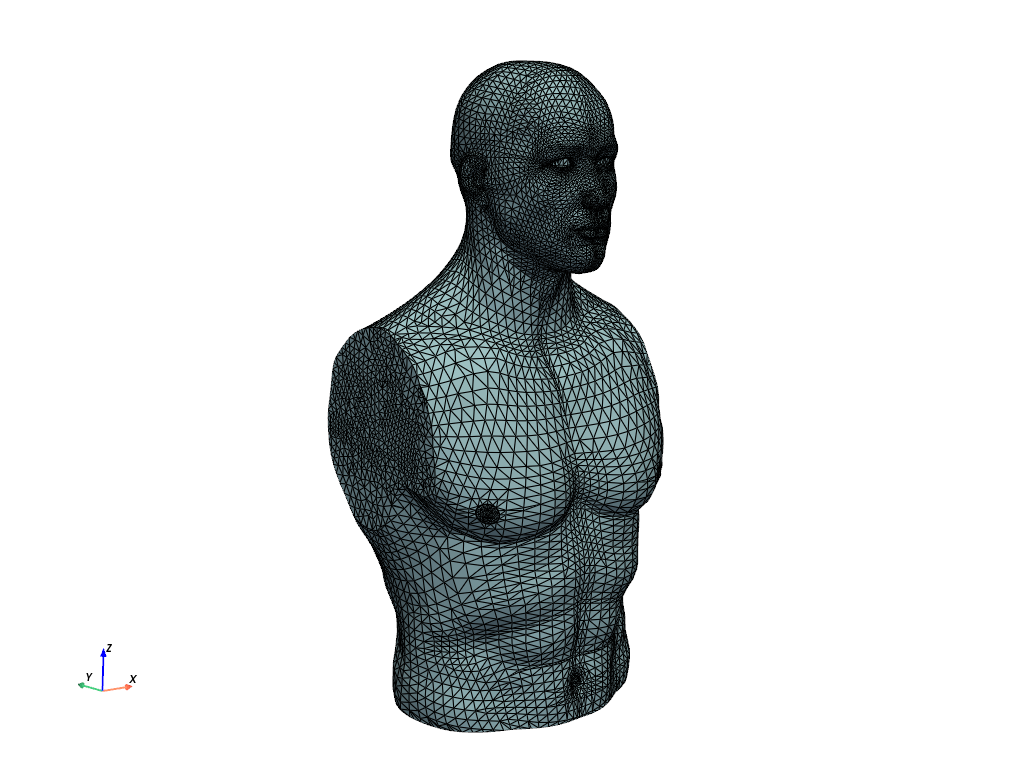# Torso¶

Repair the torso mesh where it was extracted and subtle holes along complex parts of the mesh

```# sphinx_gallery_thumbnail_number = 2
import pyvista as pv
import pymeshfix as mf
from pyvista import examples

print(mesh)
```

Out:

```PolyData (0x7fd4b82a0768)
N Cells:      12448
N Points:     12015
X Bounds:     -1.680e+02, 1.720e+02
Y Bounds:     -1.624e+02, 1.320e+02
Z Bounds:     -6.442e+02, 9.083e+01
N Arrays:     0
```
```cpos = [(-1053., -1251., 83.),
(2., -15., -276.),
(0.12, 0.18, 1)]

mesh.plot(color=True, show_edges=True, cpos=cpos)
```Appy a triangle filter to ensure the mesh is simply polyhedral

```meshfix = mf.MeshFix(mesh.tri_filter())
holes = meshfix.extract_holes()
```

Outline the holes in the mesh

```p = pv.Plotter()
p.enable_eye_dome_lighting() # helps with depth perception
p.camera_position = cpos
p.show()
```Emphasize the hole near the ear - this needs to be repaired so that the mesh is absolutely water tight.

```cpos_ear = [(180., -206., 17.),
(107., -122.9, -11.9),
(-0.13, 0.22, 0.96)]

p = pv.Plotter()
p.enable_eye_dome_lighting() # helps with depth perception
p.camera_position = cpos_ear
p.show()
```Perfrom the mesh repair

```meshfix.repair(verbose=True)
```

Out:

```Removed 6 small components
Patching holes...
Patched 5 holes
Fixing degeneracies and intersections
```

Show the repaired mesh

```meshfix.mesh.plot(cpos=cpos, eye_dome_lighting=True)
```Total running time of the script: ( 0 minutes 27.343 seconds)

Gallery generated by Sphinx-Gallery